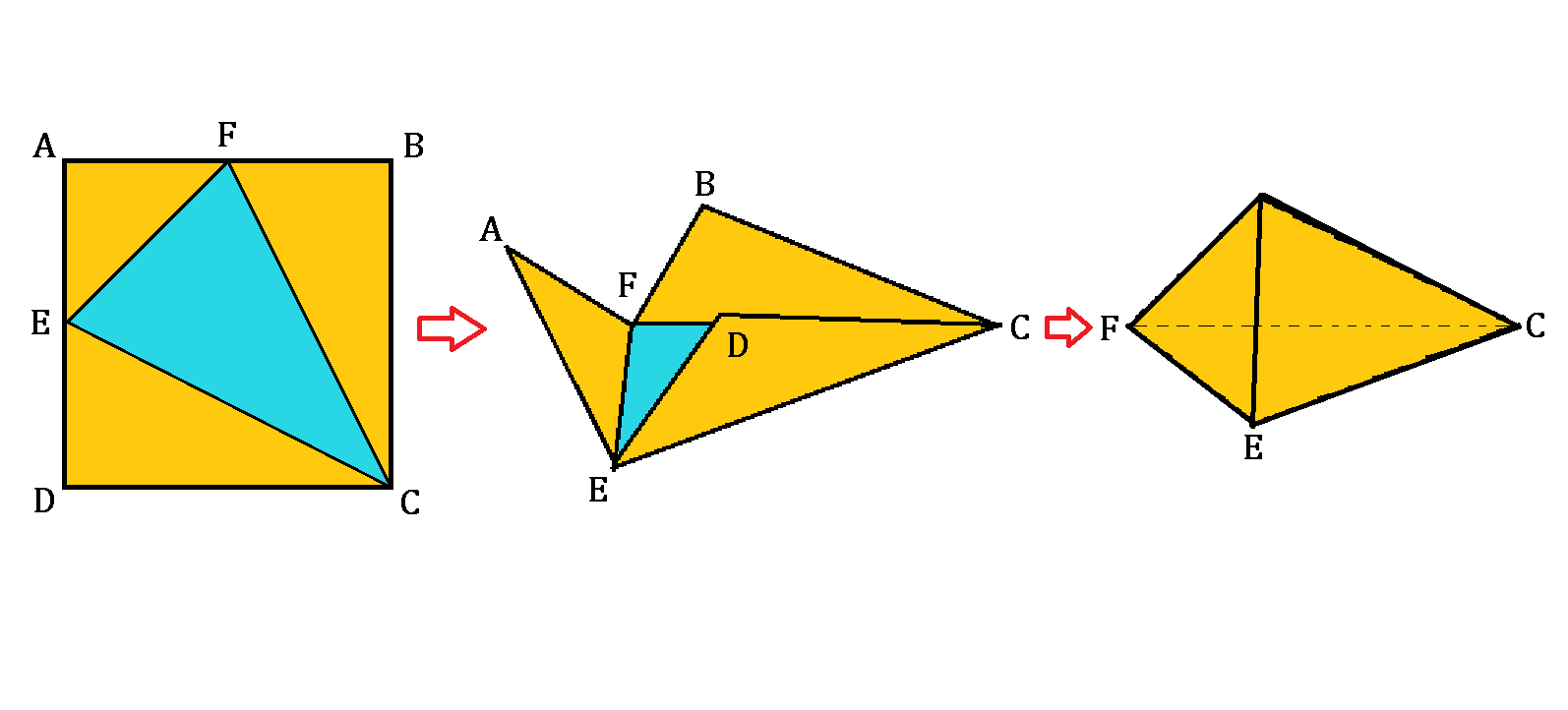# Raising A Pyramid

Geometry Level 4A square $ABCD$ has points $E$ and $F$ as its midpoints on $AD$ and $AB$, respectively. The square is then folded such that the vertices $A$, $B$, and $D$ joined together become a new vertex of the pyramid with triangular base $EFC$.

If the square has a side length of $6\text{ cm}$, what is the volume of the pyramid (in $\text{cm}^3$)?

×